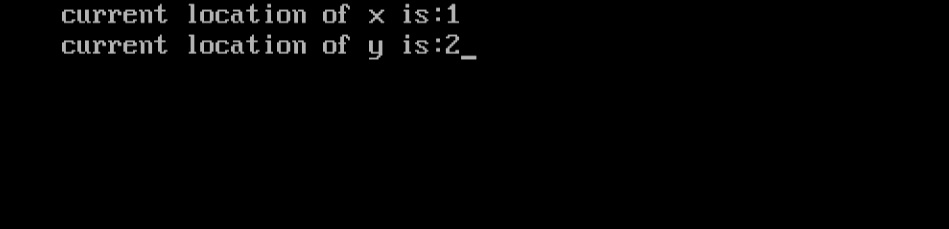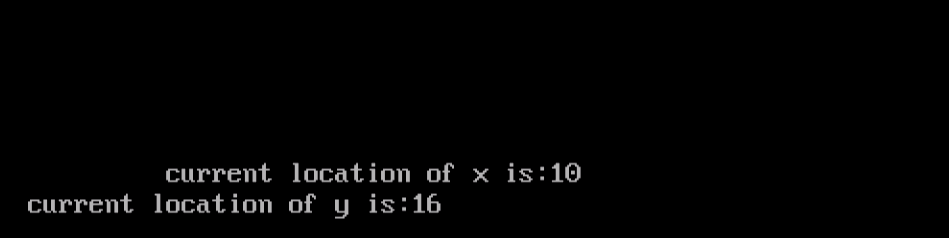# How to get the current position of cursor from output screen in C?

• Difficulty Level : Easy
• Last Updated : 06 Mar, 2019

The given task is to get the current position of the cursor from the output screen in C.

Approach: There is a predefined function wherex() in C language that returns the x coordinate of the cursor in the current output screen. And wherey() function that returns the y coordinate of the cursor in current output screen. Both the functions are defined in conio.h headerfile.

• wherex(): This method returns the horizontal position of the cursor.

Syntax:

`int wherex();`

Parameters: This method do not accepts any parameters.

Return Value: This method returns an integer value in range 1 to 80, or as per the system’s screen size.

• wherey(): This method returns the vertical position of the cursor.

Syntax:

`int wherey();`

Parameters: This method do not accepts any parameters.

Return Value: This method returns an integer value in range 1 to 50, or as per the system’s screen size.

Note: This code is according to turbo C.

Example 1:

 `// C program to get the current``// cursor position from output screen`` ` `#include ``#include `` ` `void` `main()``{``    ``clrscr();`` ` `    ``// print current location of x.``    ``printf``(``"current location of x is:%d\n"``, wherex());`` ` `    ``// print the current location of y.``    ``print(``"currentlocation of y is:%d"``, wherey());`` ` `    ``getch();``}`

Output:Example 2:

 `// C program to get the current``// cursor position from output screen`` ` `#include ``#include `` ` `void` `main()``{``    ``clrscr();`` ` `    ``// takes the cursor to given coordinates``    ``// here at (10, 15).``    ``gotoxy(10, 15);`` ` `    ``// print current location of x.``    ``printf``(``"current location of x is:%d\n"``, wherex());`` ` `    ``// print the current location of y.``    ``print(``"currentlocation of y is:%d"``, wherey());`` ` `    ``getch();``}`

Output:References:

My Personal Notes arrow_drop_up